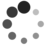# [c-faq] 2.10-designated initializer

by PingboWen of TinyLab.org 2013/08/24

``````struct test p= {
.initial=3;
.ptr=xx;
};
``````

``````int a={1,2,3,4,5,6};
``````

``````int a={  = 4,  = 8 };
``````

``````int a={0,0,4,0,0,8};
``````

``````int array[]={[0 ... 9] = 1, [55 ... 99] = 4, =23};
``````

int array={=3,5,=7};

``````struct point {
int x;
int y;
};
``````

``````struct point p1={.y=3, .x=6};
``````

``````struct point p1={6,3};
``````

``````struct point p1={y:3, x:6};
``````

``````struct point parray={.y=4,.x=1,.x=6};
``````

``````union foo{ int x; double y;};
``````

``````union foo f={.y=5};
``````

``````#include <stdio.h>
struct point {
int x;
int y;
};

int main(int argc,char *argv[])
{
int a={ =29, =15};

int widths[]={ [0 ... 9]=1, [10 ... 99]=2, =3};

struct point p1={.x=23, .y=55};

struct point p2={x:43, y:12};

int b={ =23,44,=11};

// struct point parray={.y=33, .x=88, .x=20, .y:66};

return 0;
}
``````